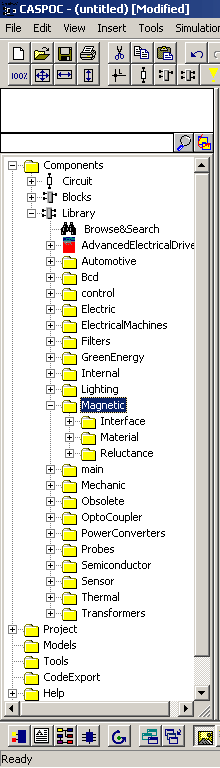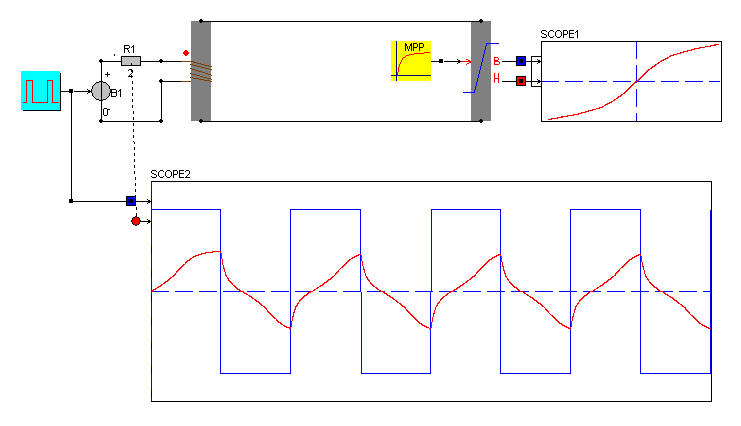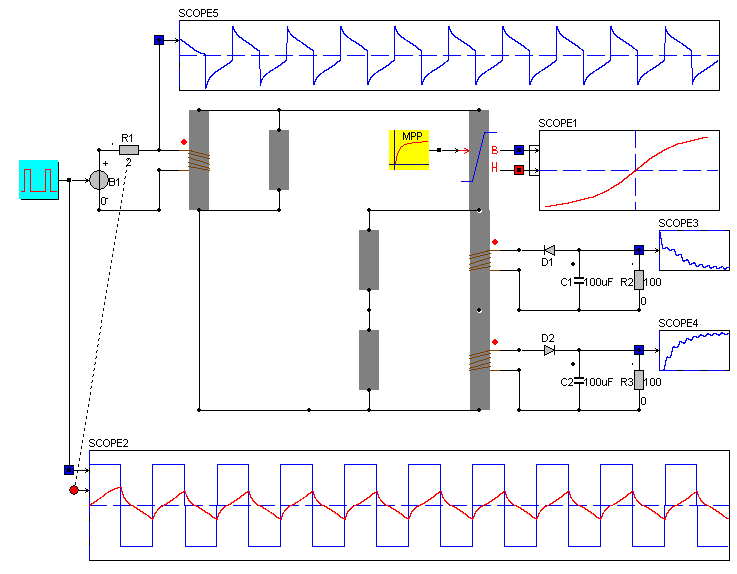• Introduction
• What is in this manual
• What is Caspoc
• User interface
• Introduction
• Starting
• Simulation
• Editing
• Viewing and printing
• Getting Started
• Basic editing
• Simulation in the time domain
• Basic User Interface Topics
• Editing
• Simulation
• Viewing
• Library
• Reports
• Project management
• Circuit and Block Diagram Components
• Introduction
• Cscript and user defined functions
• Component parameters
• Modeling Topics
• Introduction
• Power Electronics
• Semiconductors
• Electrical Machines
• Electrical drives
• Power Systems
• Mechanical Systems
• Thermal Systems
• Magnetic Circuits
• Green Energy
• Coupling to FEM
• Experimenter
• Analog hardware description language
• Embedded C code Export
• Coupling to Spice
• Small Signal Analysis
• Matlab coupling
• Tips and tricks
• Appendices

## Magnetic circuit modeling overview.

Magnetic circuit modeling is required to simulate the non-linear magnetic behavior of coupled inductors, transformers and actuators. The magnetic circuit replaces coupled inductors when the saturation of the core influences the overall behavior of the circuit. Also using the magnetic circuit, it is very easy to couple more inductors to a magnetic circuit. For example in a isolated switched mode power supply the primairy, secondary and other windings can be defined as well as size of the core and the material properties. The library components can be found in the section Components/Library/MagneticSaturation is modeled by applying the magnetic saturation properties to the magnetic circuit components. The simulation below shows the saturation of an MPP core when driven by a square wave signal.The scopes in the figure above show the electrical and magnetic results from the simulation. Scope 2 shows the driving voltage with the coil current and scope 1 shows the BH-curve for the magnetic component. The B and H outputs from the magnetic circuit are only available for displaying in a scope.

The simulation below shows a magnetic circuit with 3 windings and leakage.